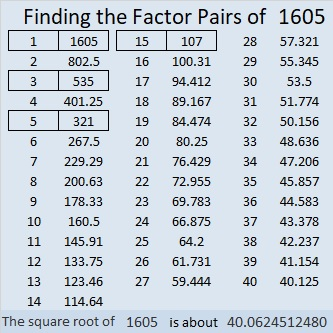# 1605 and Another Big Level One

### Today’s Puzzle:

Many of the clues in this puzzle do not appear in a 1 to 10 puzzle or a 1 to 12 puzzle. Can you find all the factors anyway? Solving this puzzle can help you solve any other Find the Factors 1 -14 puzzles.Print the puzzles or type the solution in this excel file: 14 Factors 1604-1612.

### Factors of 1605:

• 1605 is a composite number.
• Prime factorization: 1605 = 3 × 5 × 107.
• 1605 has no exponents greater than 1 in its prime factorization, so √1605 cannot be simplified.
• The exponents in the prime factorization are 1, 1, and 1. Adding one to each exponent and multiplying we get (1 + 1)(1 + 1)(1 + 1) = 2 × 2 × 2 = 8. Therefore 1605 has exactly 8 factors.
• The factors of 1605 are outlined with their factor pair partners in the graphic below.### More about the Number 1605:

1605 is the hypotenuse of a Pythagorean triple:
963-1284-1605, which is (3-4-5) times 321.

1605 is the difference of two squares four different ways:
803² – 802² = 1605,
269² – 266² = 1605,
163² – 158² = 1605, and
61² – 46² = 1605.